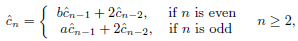##### A Weibull-Gompertz Makeham Distribution with Properties and Application to Cancer Data

Peter O. Koleoso, Angela U. Chukwu

Journal of Advances in Mathematics and Computer Science, Page 1-17
DOI: 10.9734/jamcs/2019/v34i530223

The article presents an extension of the Gompertz Makeham distribution using the Weibull-G family of continuous probability distributions proposed by Tahir et al. (2016a). This new extension generates a more flexible model called Weibull-Gompertz Makeham distribution. Some statistical properties of the distribution which include the moments, survival function, hazard function and distribution of order statistics were derived and discussed. The parameters were estimated by the method of maximum likelihood and the distribution was applied to a bladder cancer data. Weibull-Gompertz Makeham distribution performed best (AIC = -6.8677, CAIC = -6.3759, BIC = 7.3924) when compared with other existing distributions of the same family to model bladder cancer data.

##### Sum Formulas for Generalized Fifth-Order Linear Recurrence Sequences

Yüksel Soykan

Journal of Advances in Mathematics and Computer Science, Page 1-14
DOI: 10.9734/jamcs/2019/v34i530224

In this paper, closed forms of the summation formulas for generalized Pentanacci numbers are presented. Then, some previous results are recovered as particular cases of the present results. As special cases, we give summation formulas of Pentanacci, Pentanacci-Lucas, fifth order Pell, fifth order Pell-Lucas, fifth order Jacobsthal and fifth order Jacobsthal-Lucas sequences. We present the proofs to indicate how these formulas, in general, were discovered. In fact, all the listed formulas of the special cases of of the main theorems may be proved by induction, but that method of proof gives no clue about their discovery.

##### Performance of the New Ridge Regression Parameters

Mowafaq Muhammed Al-Kassab, Mohammed Qasim Al-Awjar

Journal of Advances in Mathematics and Computer Science, Page 1-9
DOI: 10.9734/jamcs/2019/v34i530225

A new approach is presented to find the ridge parameter k when the multiple regression model suffers from multicollinearity. This approach studied two cases, for the value k, scalar, and matrix. A comparison between this proposed ridge parameter and other well-known ridge parameters evaluated elsewhere, in terms of the mean squares error criterion, is given. Examples from several research papers are conducted to illustrate the optimality of this proposed ridge parameter k.

##### A New Generalization of Jacobsthal Lucas Numbers (Bi-Periodic Jacobsthal Lucas Sequence)

Sukran Uygun, Evans Owusu

Journal of Advances in Mathematics and Computer Science, Page 1-13
DOI: 10.9734/jamcs/2019/v34i530226

In this study, we bring into light a new generalization of the Jacobsthal Lucas numbers, which shall also be called the bi-periodic Jacobsthal Lucas sequence aswith initial conditions $$\ \hat{c}_{0}=2,\ \hat{c}_{1}=a.$$

The Binet formula as well as the generating function for this sequence are given. The convergence property of the consecutive terms of this sequence is examined after which the well known Cassini, Catalan and the D'ocagne identities as well as some related summation formulas are also given.

##### Testing the Robustness of Linear Programming* Using a Diet Problem on a Multi-Shop System

Sampson Takyi Appiah, Bernard Atta Adjei, Dominic Otoo, Eric Okyere

Journal of Advances in Mathematics and Computer Science, Page 1-12
DOI: 10.9734/jamcs/2019/v34i530222

Time, raw materials and labour are some of the nite resources in the world. Due to this, Linear Programming* (LP) is adopted by key decision-markers as an innovative tool to wisely consume these resources. This paper test the strength of linear programming models and presents an optimal solution to a diet problem on a multi-shop system formulated as linear, integer linear and mixed-integer linear programming models. All three models gave different least optimal values, that is, in linear programming, the optimal cost was GHS15.26 with decision variables being continuous (R+) and discrete (Z+). The cost increased to GHS17.50 when the models were formulated as mixed-integer linear programming with decision variables also being continuous (R+) and discrete (Z+) and lastly GHS17.70 for integer linear programming with discrete (Z+) decision variables. The difference in optimal cost for the same problem under different search spaces sufficiently establish that, in programming, the search space undoubtedly affect the optimal value. Applications to most problems like the diet and scheduling problems periodically require both discrete and continuous decision variables. This makes integer and mixed-integer linear programming models also an effective way of solving most problems. Therefore, Linear Programming* is applicable to numerous problems due to its ability to provide different required solutions.